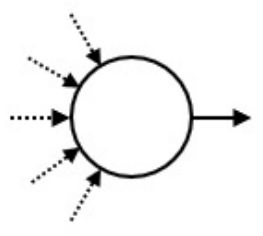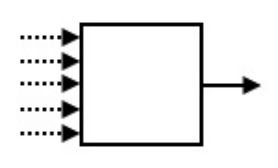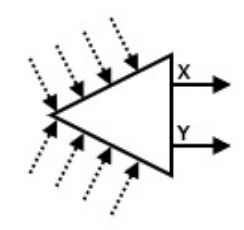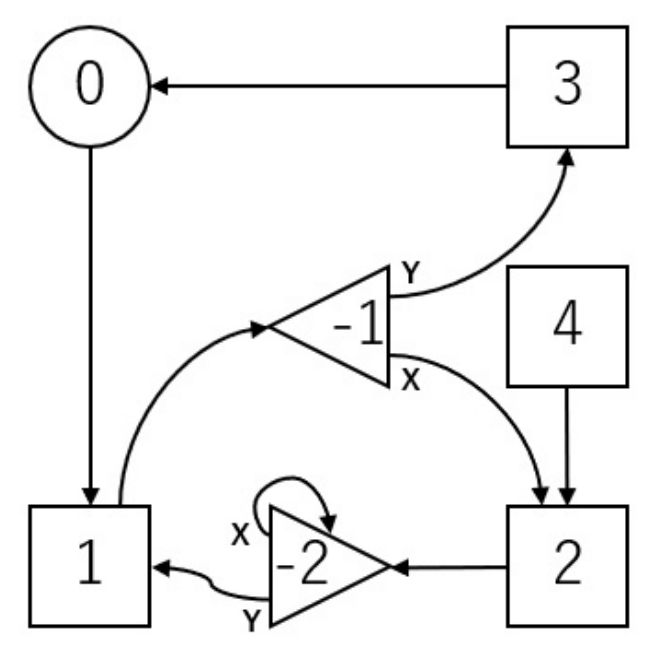시간 제한메모리 제한제출정답맞힌 사람정답 비율
1 초 512 MB55302953.704%

## 문제

A mechanical doll is a doll which automatically repeats a specific sequence of motions. In Japan, many mechanical dolls have been created since ancient times.

The motions of a mechanical doll are controlled by a circuit that consists of devices. The devices are connected with tubes. Each device has one or two exits, and can have arbitrarily many (possibly zero) entrances. Each tube connects an exit of a device to an entrance of the same or another device. Exactly one tube is connected to each entrance, and exactly one tube is connected to each exit.

To describe how the doll makes motions, consider a ball that is placed on one of the devices. The ball travels through the circuit. At each step of the travel, the ball leaves the device using one of its exits, travels along the tube connected to the exit and enters the device at the other end of the tube.

There are three types of devices: origin, trigger, and switch. There are exactly one origin, M triggers, and S switches (S can be zero). You must decide the value of S. Each device has a unique serial number.

The origin is the device where the ball is initially placed. It has one exit. Its serial number is 0.A trigger causes the doll to make a specific motion whenever the ball enters it. Every trigger has one exit. The serial numbers of the triggers are from 1 through M.Each switch has two exits, which are called 'X' and 'Y'. The state of a switch is either 'X' or 'Y'. After the ball enters a switch, it leaves the switch using the exit given by the current state of the switch. After that, the switch changes its state to the opposite one. Initially, the state of every switch is 'X'. The serial numbers of the switches are from -1 through -S.You are given the number of triggers M. You are also given a sequence A of length N, each of whose element is a serial number of a trigger. Each trigger might appear some (possibly zero) times in the sequence A. Your task is to create a circuit that satisfies the following conditions:

• The ball returns to the origin after some steps.
• When the ball first returns to the origin, the state of every switch is 'X'.
• The ball first returns to the origin after entering triggers exactly N times. The serial numbers of the triggers, in the order that they are entered, are A0, A1, ..., AN-1.
• Let P be the total number of state changes of all switches caused by the ball before the ball first returns to the origin. The value of P doesn't exceed 20,000,000.

At the same time, you don't want to use too many switches.

## 구현

You should implement the following procedure.

create_circuit(int M, int[] A)
• M: the number of triggers.
• A: an array of length N, giving the serial numbers of the triggers the ball needs to enter, in the order they are to be entered.
• This procedure is called exactly once.
• Note that the value of N is the length of the array A, and can be obtained as indicated in the implementation notice.

answer(int[] C, int[] X, int[] Y)
• C: an array of length M + 1. The exit of the device i (0 ≤ iM) is connected to the device C[i].
• X, Y: arrays of the same length. The length S of these arrays is the number of the switches. For the switch -j (1 ≤ jS), its exit 'X' is connected to the device X[j - 1] and its exit 'Y' is connected to the device Y[j - 1].
• Every element of C, X, and Y must be an integer between -S and M, inclusive.
• S must be at most 400,000.
• This procedure must be called exactly once.
• The circuit represented by C, X, and Y must satisfy the conditions in the problem statement.

If some of the above conditions are not satisfied, your program is judged as Wrong Answer. Otherwise, your program is judged as Accepted and your score is calculated by S (see Subtasks).

## 예시

Let M = 4, N = 4, and A = [1, 2, 1, 3]. The grader calls create_circuit(4, [1, 2, 1, 3]).The above figure shows a circuit, which is described by a call answer([1, -1, -2, 0, 2], [2, -2], [3, 1]). The numbers in the figure are the serial numbers of the devices.

Two switches are used. Thus S = 2.

Initially, the states of the switches -1 and -2 are both 'X'.

The ball travels as follows:

0 → 1 → -1 → 2 → -2 → -2 → 1 → -1 → 3 → 0

• When the ball first enters the switch -1, its state is 'X'. Hence, the ball travels to the trigger 2. Then the state of the switch -1 is changed to 'Y'.
• When the ball enters the switch -1 for the second time, its state is 'Y'. Hence, the ball travels to the trigger 3. Then the state of the switch -1 is changed to 'X'.

The ball first returns to the origin, having entered the triggers 1, 2, 1, 3. The states of the switches -1 and -2 are both 'X'. The value of P is 4. Therefore, this circuit satisfies the conditions.

## 제한

• 1 ≤ M ≤ 100,000
• 1 ≤ N ≤ 200,000
• 1 ≤ AkM (0 ≤ kN - 1)

## 서브태스크

번호 배점 제한
1 2

For each i (1 ≤ iM), the integer i appears at most once in the sequence A0, A1, ..., AN-1.

2 4

For each i (1 ≤ iM), the integer i appears at most twice in the sequence A0, A1, ..., AN-1.

3 10

For each i (1 ≤ iM), the integer i appears at most 4 times in the sequence A0, A1, ..., AN-1.

4 10

N = 16

5 18

M = 1

6 56

## 점수

For each test case, if your program is judged as Accepted, your score is calculated according to the value of S:

• If SN + log2N, you gain the full score for the test case.
• For each test case in Subtasks 5 and 6, if If SN + log2N, you gain a partial score. The score for the test case is 0.5 + 0.4 × ((2N - S)/(N - log2N))2, multiplied by the score assigned to the subtask.
• Otherwise, the score is 0.

Note that your score for each subtask is the minimum of the scores for the test cases in the subtask.

## 샘플 그레이더

The sample grader reads the input from the standard input in the following format.

• line 1: M N
• line 2: A0 A1 ... AN-1

The sample grader produces three outputs.

First, the sample grader outputs your answer to a file named out.txt in the following format.

• line 1: S
• line 2 + i (0 ≤ iM): C[i]
• line 2 + M + j (1 ≤ jS): X[j - 1] Y[j - 1]

Second, the sample grader simulates the moves of the ball. It outputs the serial numbers of the devices the ball entered in order to a file named log.txt.

• If your program is judged as Accepted, the sample grader prints and in the following format Accepted: S P.
• If your program is judged as Wrong Answer, it prints Wrong Answer: MSG. The meaning of MSG is as follows:
• answered not exactly once: The procedure answer is called not exactly once.
• wrong array length: The length of C is not M + 1, or the lengths of X and Y are different.
• over 400000 switches: S is larger than 400,000.
• wrong serial number: There is an element of C, X, or Y which is smaller than -S or larger than M.
• over 20000000 inversions: The ball doesn't return to the origin within 200,000,000 state changes of the switches.
• state 'Y': There is a switch whose state is 'Y' when the ball first returns to the origin.
• wrong motion: The triggers which cause motions are different from the sequence A.

Note that the sample grader might not create out.txt and/or log.txt when your program is judged as Wrong Answer.

## 출처• 문제를 만든 사람: Tomasz Idziaszek

## 제출할 수 있는 언어

C++17, C++14, C++20, C++14 (Clang), C++17 (Clang), C++20 (Clang)

## 채점 및 기타 정보

• 예제는 채점하지 않는다.